## Hypersphere Packing

Draw unit-spheres in an-D space centered at allcoordinates. Then place an additional Hypersphere at the origin tangent to the other Hyperspheres. Then the central Hypersphere is contained inside the Hypercube with Vertices at the centers of the other spheres forbetween 2 and 8. However, for, the central Hypersphere just touches the Hypercube of centers, and for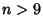, the Hypersphere is partially outside the hypercube. This can be seen by finding the distance from the origin to the center of one of the Hyperspheres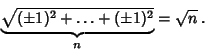The radius of the central sphere is therefore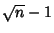. The distance from the origin to the center of the bounding hypercube is always 2 (two radii), so the center Hypersphere is tangent when, or, and outside for.

The analog of face-centered cubic packing is the densest lattice in 4- and 5-D. In 8-D, the densest lattice packing is made up of two copies of face-centered cubic. In 6- and 7-D, the densest lattice packings are cross-sections of the 8-D case. In 24-D, the densest packing appears to be the Leech Lattice. For high dimensions (-D), the densest known packings are nonlattice. The densest lattice packings in-D have been rigorously proved to have Packing Density 1,,,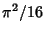,,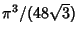,, and(Finch).

The largest number of unit Circles which can touch another is six. For Spheres, the maximum number is 12. Newtonconsidered this question long before a proof was published in 1874. The maximum number of hyperspheres that can touch another in-D is the so-called Kissing Number.

Finch, S. Favorite Mathematical Constants.'' http://www.mathsoft.com/asolve/constant/hermit/hermit.html